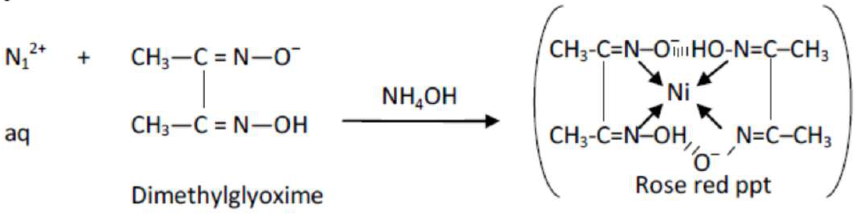# Given below are two statements :Question:

Given below are two statements :

Statement $\mathrm{I}$ : The identification of $\mathrm{Ni}^{2+}$ is carried out by dimethyl glyoxime in the presence of $\mathrm{NH}_{4} \mathrm{OH}$

Statement II : The dimethyl glyoxime is a bidentate neutral ligand.

In the light of the above statements, choose the correct answer from the options given below :

1. Both statement I and statement II are true

2. Both statement I and statement II are false

3. Statement I is false but statement II is true

4. Statement $\mathrm{I}$ is true but statement $\mathrm{II}$ is false

Correct Option: , 4

Solution:Dimethyl glyoxime is a negative bidentate legend.